## Icosahedron

A Platonic Solid (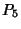) with 12 Vertices, 30 Edges, and 20 equivalent Equilateral Triangle faces. It is described by the Schläfli Symbol. It is also Uniform Polyhedronand has Wythoff Symbol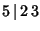. The icosahedron has the Icosahedral Groupof symmetries.

A plane Perpendicular to aaxis of an icosahedron cuts the solid in a regular Decagonal Cross-Section (Holden 1991, pp. 24-25).

A construction for an icosahedron with side lengthplaces the end vertices at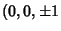) and the central vertices around two staggered Circles of Radii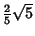and heights, giving coordinates(1)

for, 1, ..., 4, where all the plus signs or minus signs are taken together. Explicitly, these coordinates are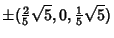(2)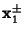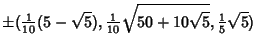(3)(4)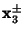(5)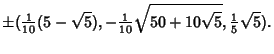(6)

By a suitable rotation, the Vertices of an icosahedron of side length 2 can also be placed at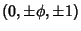,, and, whereis the Golden Ratio. These points divide the Edges of an Octahedron into segments with lengths in the ratio.

The Dual Polyhedron of the icosahedron is the Dodecahedron. There are 59 distinct icosahedra when each Triangle is colored differently (Coxeter 1969).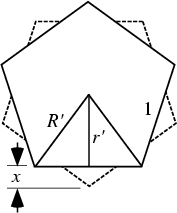To derive the Volume of an icosahedron having edge length, consider the orientation so that two Vertices are oriented on top and bottom. The vertical distance between the top and bottom Pentagonal Dipyramids is then given by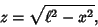(7)

where(8)

is the height of an Isosceles Triangle, and the Sagittaof the pentagon is(9)

giving(10)

Plugging (8) and (10) into (7) gives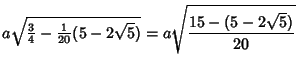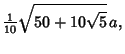(11)

which is identical to the radius of a Pentagon of side. The Circumradius is then(12)

where(13)

is the height of a Pentagonal Dipyramid. Therefore,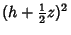(14)

Taking the square root gives the Circumradius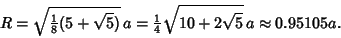(15)(16)

The square of the Interradius is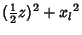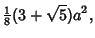(17)

so(18)

The Area of one face is the Area of an Equilateral Triangle(19)

The volume can be computed by taking 20 pyramids of height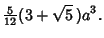(20)

Apollonius showed that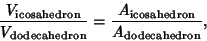(21)

where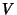is the volume andthe surface area.

See also Augmented Tridiminished Icosahedron, Decagon, Dodecahedron, Great Icosahedron, Icosahedron Stellations, Metabidiminished Icosahedron, Tridiminished Icosahedron, Trigonometry Values Pi/5

References

Coxeter, H. S. M. Introduction to Geometry, 2nd ed. New York: Wiley, 1969.

Davie, T. The Icosahedron.'' http://www.dcs.st-and.ac.uk/~ad/mathrecs/polyhedra/icosahedron.html.

Holden, A. Shapes, Space, and Symmetry. New York: Dover, 1991.

Klein, F. Lectures on the Icosahedron. New York: Dover, 1956.

Pappas, T. The Icosahedron & the Golden Rectangle.'' The Joy of Mathematics. San Carlos, CA: Wide World Publ./Tetra, p. 115, 1989.